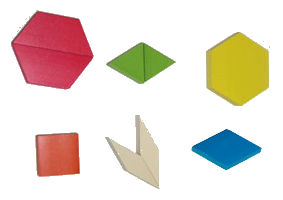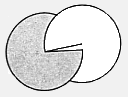# Fractional number values development, instructional ideas, sample activities, and assessment to represent fractions and decimals

Page Overview:

This page includes information on tasks to facilitate the development of fractions: fractions as equal parts of a whole, fractions as equal subset of a group, and equivalent fractions to hundredths. The use of fraction circles, fraction disks, paper folding, area models, measurement models, and number lines to represent fractional values.
Also see: number lines background information, and Fraction concepts & misconceptions

Young students can explore fractions as  equal parts of a whole visually before they  conserve area if the areas are identical in shape as the area of the whole. For example: half of a square in the shape of a rectangle or triangle which is half of a square as the whole or unit.

Activities where students explore different shapes and discuss which are identical, which are not, and then which parts of other shapes can fit together to make the original shape, help them conceptualize concepts of fractional values. Showing how two identical shapes can fit into a larger shape. For example: a square can be made with identical rectangles (each 1/2 of the square), two identical triangles (each 1/2 of the square). Pattern blocks can be manipulated to represent this as well as cutting paper, or folding paper to verify equal sizes. Those that are and can be used to complete a larger shape entirely can be identified as fractional parts (1/2, 1/3, & 1/4).

Similar strategies can be used with older students as they develop spatial abilities to rotate and flip shapes and objects to identify fractional parts in more complicated representations.

## Fraction models

### Area model

Fraction as equal parts of a whole.

Give fractional strips and ask to fold them into different fractional values (1/2 & 1/3). Verify with folding.

Give fraction circles and ask to fold or color them into different fractional values (1/2, 1/4, 3/4, 1/3, 2/3). Verify with folding or counting equal fractional parts.

Challenge with examples of fractional parts of shapes to identify specific fractional parts (1/2, ...). A whole shape and parts of the shape of different sizes. Verify by folding or placing fractional parts on whole.

• Ask. Describe which shape is one-half of the square. If students don't comment on the portion size of each piece, ask them questions about the size of each part. Have them verify their answers by folding the square or strips in halves.

Worksheet samples:

Scoring guide or rubric

### Area & volume models

Fraction values with Pattern Blocks & Cuisenaire rods (area? & volume models)Give students a variety of pattern blocks or Cuisenaire rods (see chart below) and ask them to show 1/2, 1/3, 2/3, 3/4, and 1 1/2 .Have them verify their answers by arranging equal rods or blocks to match the unit block or rod. Scoring guide or rubric

Worksheet samples:

If students can't complete the task on their own, prompt them by giving them a block to use as the value of one and ask if they can show the fractional part of it. (1/2, 1/3, 2/3, 3/4, and 1 1/2) Scoring guide or rubricFraction Rods
1/2 Red and white,
Red and purple,
Yellow and orange,
Light green and dark green,
1/3 Light green and blue,
Or white and light green,
Or red and dark green
2/3 Light green and blue,
Or white and light green,
Or red and dark green,
1 and 1/2 Red and white,
Red and purple,
Yellow and orange,
Light green and dark green,Fractions with Pattern blocks (area? & volume models)

Give students an assortment of Pattern blocks and ask them to show you 1/2, 1/3, 2/3, 3/4, 1 1/2. Have them verify their answers by arranging equal rods or blocks to match the unit block or rod.

Fraction Pattern Blocks
1/2

Yellow hexagon and red trapezoid

Or blue parallelogram and red trapezoid

1/3

Red trapezoid and green triangles,

Or yellow hexagon and blue parallelogram

2/3

Red trapezoid and green triangles,

Or yellow hexagon and blue parallelogram

1 and 1/2

Yellow hexagon and red trapezoid,

Or blue parallelogram and red trapezoid

Scoring guide or rubric

### Measurement model

Fractions with length or measurement models:

• Give students a ruler and ask them to show you 1/2, 3/4, and 3/8 of an inch. Have them verify their answers by counting the number of spaces in an inch, cm, or m for the unit (inch, foot, cm, m).
• If they can not then prompt them by showing them 1/8, 1/4, and 1/2, then ask them to show you 3/4, 3/8 and 1/2.
• Fractions on number lines

Worksheet samples:

• Fraction strips
• Number lines
• Rulers - locate fractional parts of an inch: 1/2, 1/4, 1/8, 1/16, 3/8, 2/4, 3/4 ...
• Rulers - locate fractional parts of a cm and m : 1/2, 1/5, 1/10, 2/4, 2/5, 2/10, 3/10, ...

Scoring guide or rubric

### Sub sets

Fractions as subsets of a group (set):

Give students collections of objects and ask them to show you 1/2, 1/3, 1/4, 1/5, 1/6, 2/4, 3/4, 3/6, 2/5, 3/10 ... Have them verify their answers by arranging equal subsets for the fractional number of subsets in the group.

If they can't prompt them by dividing the set into two groups and asking what is 1/2.

Five groups and ask what is 2/5.

Worksheet sample:

Scoring guide or rubric

## Equivalent Fractions

Give students a fraction wheel and ask them to explain what different parts are equivalent. Have them verify their answers by showing the equal the fractional number of fractional parts of in the circle.

Ask. Can you show equivalent fractions.

If they can't prompt them by showing them that the inside circle is 1/4, then ask them if that helps them know what the middle section and outer section is. Then ask what that has to do with equivalent fractions.

Worksheets & samples:

Scoring guide or rubric

## Comparing fractions

### Comparing fractions as equal, greater, or less:

Ask students how they would arrange 1/2, 1/3, 1/4, and 2/3 in order and how they know they are right. Shaded values on equal sized fraction strips and number lines can be compared by lengths. Others by areas or volumes if the units are the same.

Worksheet sample:

### Using one

Know what represents one and the different parts of a fraction (numerator & denominator).

Samples:

• Use any of the object or shapes to ask. Use these objects (collection of Cuisenaire rods (W, R, G, D, B, ... ) and show 1/3. What does the one (numerator) represent? What does the three (denominator) represent? What represents a whole?
• Next give them an object, that can represent 2/10, (red Cuisenaire rod if O (orange) is one) and say. If this (red rod) is 1/5, what is one (O).
• Use a shape and have learners see how many ways they can divide it into fractional parts. Example: How many different ways can you divide a square into eights? Sample

# Activity sheets

## Fractional parts of a shape: Square and Parts

Find which shapes are 1/2 (one-half) of the top square.## Cuisenaire Rods for Fractional partsWhat fractional parts can you illustrate for the different rods?

## Pattern blocksWhat fractional parts can you illustrate for the pattern blocks?

## Fractional parts of groups

What fractional parts are the different colors?Green

Pink

Yellow

Blue

What is one?

## Fractional parts of a circle

What fractional parts can you make in the circle?## Fraction WheelCut out each disk. Cut along the radius line. Slide each disk inside the other. Rotate the wheels to show different fractional parts of a circle.## Fractional parts of a square challenge

What fractional parts do the different shapes in the square represent?## Number lines for fractional parts

### Number line with 12 spaces

What fractional parts can you represent on the number line?### Number line with 36 spaces

What fractional parts can you represent on the number line?### One inch ruler for fractional parts

What equivalent fractional parts can you represent on the first inch of an enlarged ruler?## Number lines for decimal equivalents

What decimal parts can you represent on this enlarged centimeter or reduced meter?## Fraction strips: 10 & 20

What fractional parts can you represent with a strip?What fractional parts can you represent on the strips if one strip is 1/2 or two strips =1?## Hundred chart

What fractional parts can you represent on the square?## Hundred charts

Make fractional parts equivalent to 1ooths (.01).## Sample of different ways to divide a square into eights

Challenge. How many different ways can you divide a square into eights?